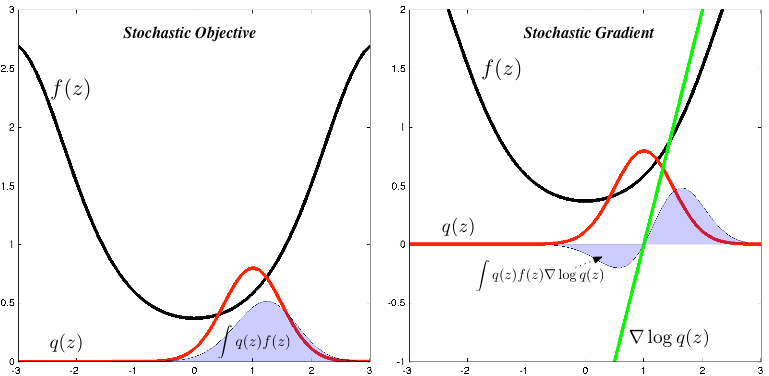# Machine Learning Trick of the Day (5): Log Derivative Trick

Machine learning involves manipulating probabilities. These probabilities are most often represented as normalised-probabilities or as log-probabilities. An ability to shrewdly alternate between these two representations is a vital step towards strengthening the probabilistic dexterity we need to solve modern machine learning problems. Today's trick, the log derivative trick, helps … Continue reading Machine Learning Trick of the Day (5): Log Derivative Trick# Machine Learning Trick of the Day (4): Reparameterisation Tricks

Our ability to rewrite statistical problems in an equivalent but different form, to reparameterise them, is one of the most general-purpose tools we have in mathematical statistics. We used reparameterisation in all the tricks we explored in this series so far: trick 1 re-expressed a … Continue reading Machine Learning Trick of the Day (4): Reparameterisation Tricks# Machine Learning Trick of the Day (3): Hutchinson's Trick

Hutchinson's estimator [cite key='hutchinson1990stochastic'] is a simple way to obtain a stochastic estimate of the trace of a matrix. This is a simple trick that uses randomisation to transform the algebraic problem of computing the trace into the statistical problem of … Continue reading Machine Learning Trick of the Day (3): Hutchinson's Trick